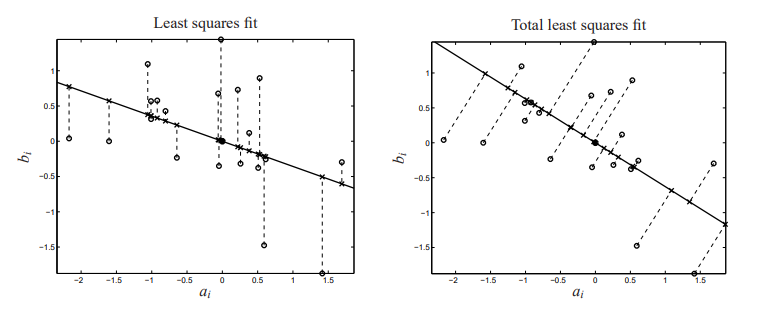## Wednesday, June 2, 2021

### Total Least Squares: nonconvex optimization

Total Least Squares (TLS) is an alternative for OLS (Ordinary Least Squares). It is a form of orthogonal regression and also deals with the problem of EIV (Errors-in-Variables).

The standard OLS model is $\color{darkblue}y = \color{darkblue}X\color{darkred}\beta + \color{darkred}\varepsilon$ where we minimize the sum-of-squares of the residuals $\min ||\color{darkred}\varepsilon||_2^2$ We can interpret $$\color{darkred}\varepsilon$$ as the error in $$\color{darkblue}y$$.

In TLS, we also allow for errors in $$\color{darkblue}X$$. The model becomes $\color{darkblue}y+\color{darkred}\varepsilon=(\color{darkblue}X+\color{darkred}E)\color{darkred}\beta$ Note that we made a sign change in $$\color{darkred}\varepsilon$$. This is pure aesthetics: to make the equation more symmetric looking. The objective is specified as $\min \> ||\left(\color{darkred}\varepsilon \> \color{darkred}E\right)||_F$ i.e. the Frobenius norm of the matrix formed by $$\color{darkred}\varepsilon$$ and $$\color{darkred}E$$. The Frobenius norm is just $||A||_F=\sqrt{\sum_{i,j}a_{i,j}^2}$ We can drop the square root from the objective (the solution will remain the same, but we got rid of a non-linear function with a possible problem near zero: the gradient is not defined there). The remaining problem is a non-convex quadratic problem which can be solved with global MINLP solvers such as Baron or with a global quadratic solver like Gurobi.

One property of TLS is that orthogonal distances are measured instead of vertical ones for OLS:Orthogonal distances for TLS (picture from )

Usually, linear TLS is solved using Singular Value Decomposition. But, here I want to try the following non-convex model:

\begin{align}\min&\sum_i \color{darkred}\varepsilon_i^2+ \sum_{i,j}\color{darkred}E_{i,j}^2\\ & \color{darkblue}y_i + \color{darkred}\varepsilon_i = \sum_j \left(\color{darkblue}X_{i,j}+\color{darkred}E_{i,j}\right) \color{darkred}\beta_j && \forall i\end{align}

#### Example

Consider the small generated data set:

----     51 PARAMETER data

y          x1          x2

i1     5.658390    0.497808    3.435376
i2    10.206082    1.545745    9.413754
i3    11.735484    1.653134    9.476257
i4    10.994595    2.886785    9.694459
i5     8.178234    2.353039   10.770926
i6    10.586022    2.768120    5.760277
i7     4.481738    2.063961    2.004087
i8     7.495135    3.542960    3.440644
i9    10.959411    2.174741    6.331947
i10    4.809324    2.802416    1.620228
i11   13.290220    3.406511   10.506181
i12    9.774514    3.560376    5.563656
i13   12.084065    3.403917    8.248807
i14    7.992731    4.481498    2.519736
i15   12.111285    3.996363    7.249193
i16   14.487092    3.970683    9.410644
i17   11.062400    3.734251    7.364956
i18    7.592865    4.753497    4.695553
i19   11.244580    3.445085    6.214768
i20    7.837413    6.782433    6.367412
i21   12.199275    4.144486    7.570009
i22   13.872630    5.454476   10.463741
i23   10.461876    5.057793    4.034925
i24   14.713012    5.672384    7.914547
i25   10.751043    4.185621    7.648005


I tried the non-convex quadratic optimization model using GAMS with different global MINLP and QCP solvers. Most solvers found the optimal solution quite quickly but had enormous problems proving optimality.

The solution for data without constant term looks like:

----     83 VARIABLE beta.L  parameter to estimate

x1 1.282931,    x2 0.844328


When we use the R model (reproduced in appendix 2 below), we note that we need to explicitly remove the constant term from the model (that is the -1 in the formula). If we would have kept the (implicit) constant term, we would have seen:

tls(Y ~ X1 + X2, data = df)
Error in tls(Y ~ X1 + X2, data = df) :
Using total least squares requires model without intercept.

The model with the constant term removed produces:

> tls(Y ~ X1 + X2 - 1, data = df)

$coefficient X1 X2 1.2829314 0.8443277$confidence.interval
2.5% lower bound 97.5% upper bound
X1        0.6508868          1.914976
X2        0.5248924          1.163763

#### Appendix 2: R code

df <- read.table(text="
id      Y        X1       X2
i1    5.658390  0.4978076  3.435376
i2   10.206082  1.5457447  9.413754
i3   11.735484  1.6531337  9.476257
i4   10.994595  2.8867848  9.694459
i5    8.178234  2.3530392 10.770926
i6   10.586022  2.7681198  5.760277
i7    4.481738  2.0639606  2.004087
i8    7.495135  3.5429598  3.440644
i9   10.959411  2.1747406  6.331947
i10   4.809324  2.8024155  1.620228
i11  13.290220  3.4065109 10.506181
i12   9.774514  3.5603761  5.563656
i13  12.084065  3.4039173  8.248807
i14   7.992731  4.4814979  2.519736
i15  12.111285  3.9963628  7.249193
i16  14.487092  3.9706833  9.410644
i17  11.062400  3.7342514  7.364956
i18   7.592865  4.7534969  4.695553
i19  11.244580  3.4450848  6.214768
i20   7.837413  6.7824328  6.367412
i21  12.199275  4.1444857  7.570009
i22  13.872630  5.4544764 10.463741
i23  10.461876  5.0577928  4.034925
i24  14.713012  5.6723841  7.914547
i25  10.751043  4.1856209  7.648005",

library(tls)
tls(Y ~ X1 + X2 - 1, data = df)


1.I tried the model with some solvers, with the following results:

Excel Solver GRG nonlinear: 5 seconds to optimality
Bonmin via OpenSolver: < 1 second to optimality
Couenne via OpenSolver: Finds optimal solution in < 1 second, but then very slow to converge towards proving optimality
Octeract: Finds optimal solution in presolve (< 3.5 seconds), but then very slow to converge towards proving optimality

In each case, I started with all variables set to zero.

1.Excel/GRG and Bonmin are local solvers, so "optimal" means locally optimal. Proving (global) optimality is surprisingly difficult for this small model.

2.That's why I tried Octeract, which claims "guaranteed global optimal solutions for any complex optimisation problem, turning insights into decisions in no time". After 10 hours, Octeract had bounds on the objective function of 18.95 and 27.84, with little change in the lower bound in the last hour. As you say, surprisingly difficult for such a small problem.